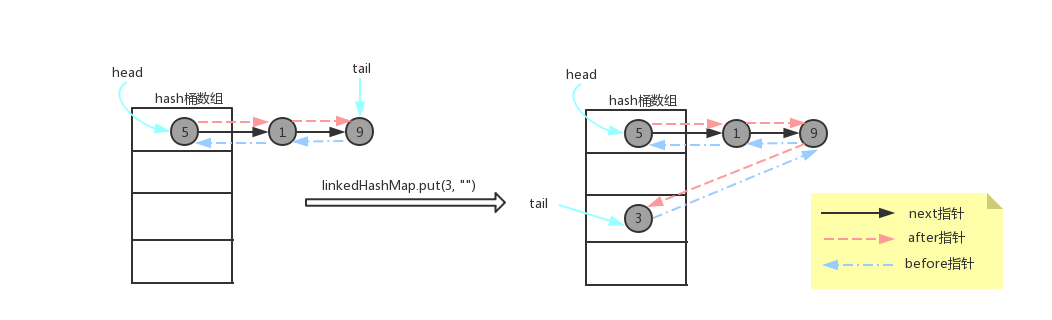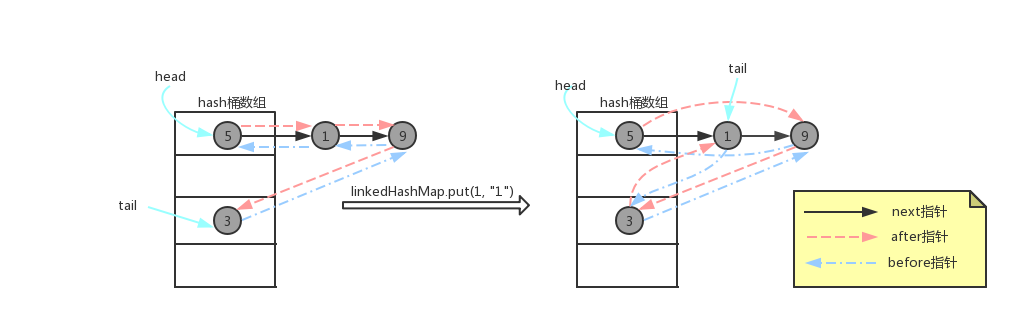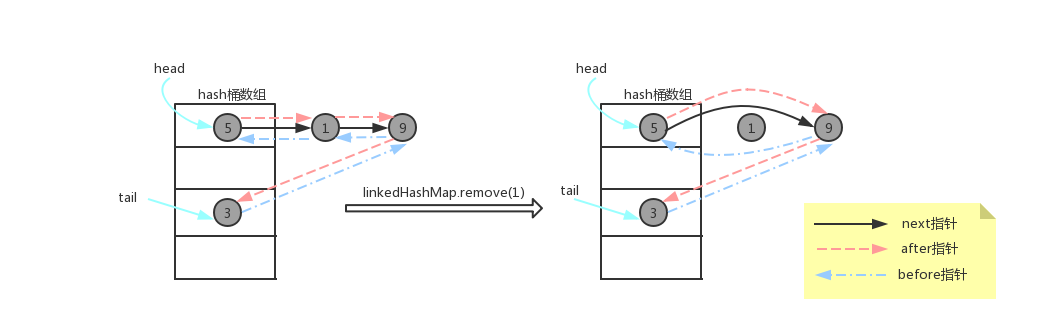coding……## 1. 调用示例

``````public static void main(String[] args) {
/*默认构造函数，按照插入顺序有序*/
System.out.println("按插入顺序有序：");
/*按插入顺序有序时，put修改操作，并不会改变顺序*/

while (iterator.hasNext()) {
System.out.println(iterator.next());
}

/*调用
boolean accessOrder)
构造函数，指定accessOrder为true时，可以按照访问顺序有序
* */
System.out.println("按访问顺序有序：");
/*1 -> 2 -> 3 -> 4*/

/*1 -> 3 -> 4 -> 2*/

/*1 -> 3 -> 2 -> 4*/

/*3 -> 2 -> 4 -> 1*/

/*3 -> 2 -> 4 -> 1 -> 5*/

while (iterator.hasNext()) {
System.out.println(iterator.next());
}
}``````

``````按插入顺序有序：
1=a
2=b
3=e
4=d

3=c
2=b
4=d
1=e
5=f``````

### 1.2 按访问有序使用场景

CPU的缓存曾经是用在超级计算机上的一种高级技术，不过现今计算机上使用的的AMD或Intel微处理器都在芯片内部集成了大小不等的数据缓存和指令缓存，通称为L1缓存；而比L1更大容量的L2缓存曾经被放在CPU外部（主板或者CPU接口卡上），但是现在已经成为CPU内部的标准组件；更昂贵的CPU会配备比L2缓存还要大的L3缓存（level 3 On-die Cache第三级高速缓冲存储器）。L1容量缓存非常小、价格非常贵、读取速度快，三级缓存容量会大一些、便宜一些、读取速度也慢一些。

``````protected boolean removeEldestEntry(Map.Entry<K,V> eldest) {
return false;
}``````

``````public class LRUCache<K, V> extends LinkedHashMap<K, V> {
private int maxSize;

public LRUCache(int maxSize){
super(16, 0.75f, true);
this.maxSize = maxSize;
}

@Override
protected boolean removeEldestEntry(Map.Entry<K, V> eldest) {
return size() > maxSize;
}

public static void main(String[] args) {
LRUCache<Integer, String> lruCache = new LRUCache<>(3);
lruCache.put(1, "a");
lruCache.put(2, "b");
lruCache.put(3, "c");
//访问1->a
//key顺序为：2 -> 3 -> 1
System.out.println(lruCache.get(1));

//插入一个新键值对，理论上，由于超过LruCache的maxSize了，所以会删除最老的键值对
//插入后，删除最老键值对前，key顺序：2 -> 3 -> 1 -> 4
//所以最老的键值对，key为2
lruCache.put(4, "d");

System.out.println(lruCache);
}
}``````

``````a
{3=c, 1=a, 4=d}``````

## 2. 方法说明

### 2.1 构造函数

S.N. 方法 说明
boolean accessOrder)

### 2.2 Map接口

S.N. 方法 说明
1 void clear() 清除Map中所有的KV映射
2 default V computeIfAbsent(K key, Function<? super K, ? extends V> mappingFunction) Java 8新方法，如果key在Map中不存在，则根据Function规则计算key对应的value值，

3 default V computeIfPresent(K key,
BiFunction<? super K, ? super V, ? extends V> remappingFunction)
Java 8新方法，如果key在Map中存在，则根据BiFunction规则通过key和对应的value

4 default V compute(K key, BiFunction<? super K, ? super V, ? extends V> remappingFunction) Java 8新方法，相当于上述两个方法的综合，根据BiFunction，通过key和对应的value

5 boolean containsKey(Object key) 判断Map中是否存在key键
6 boolean containsValue(Object value) 判断Map中是否存在value值
7 Set<Map.Entry<K, V>> entrySet() 将Map所有的键值对转换为Set集合
8 boolean equals(Object o) equals方法
9 default void forEach(BiConsumer<? super K, ? super V> action) Java 8新方法，遍历Map，通过BiConsumer处理Map中每一个键值对的key、value
10 V get(Object key) 获取Map中key对应的value
11 default V getOrDefault(Object key, V defaultValue) Java 8新方法，获取Map中key对应的value，如果key不存在，则返回defaultValue
12 int hashCode() hashCode方法
13 boolean isEmpty() 判断Map是否为空
14 Set<K> keySet() 将Map中所有的key转化为Set集合
15 default V merge(K key, V value,
BiFunction<? super V, ? super V, ? extends V> remappingFunction)

BiFunction规则通过oldValue和value计算一个newValue，替换或删除(newValue为null)原

16 V put(K key, V value); 为Map添加一个键值对
17 void putAll(Map<? extends K, ? extends V> m) 将另一个Map的所有键值对添加到该Map中
18 default V putIfAbsent(K key, V value) Java 8新方法，如果Map中key不存在，则添加一个key-value键值对
19 V remove(Object key) 从Map中移除key对应的键值对
20 default boolean remove(Object key, Object value) Java8新方法，删除key-value键值对
21 default V replace(K key, V value) Java8新方法，将Map中key对应的值替换为value
22 default boolean replace(K key, V oldValue, V newValue) Java8新方法，将Map中key-oldValue键值对的value值替换为newValue
23 default void replaceAll(BiFunction<? super K, ? super V, ? extends V> function) Java8新方法，将Map中所有的键值对的value，替换为根据BiFunction规则通过key-value

24 int size() 返回Map中EntrySet的数目
25 Collection<V> values() 返回Map中所有value组成的集合

## 3. 实现源码分析

``````public class LinkedHashMap<K,V>
extends HashMap<K,V>
implements Map<K,V>
{
/**
*/
static class Entry<K,V> extends HashMap.Node<K,V> {
Entry<K,V> before, after;
Entry(int hash, K key, V value, Node<K,V> next) {
super(hash, key, value, next);
}
}

/**
* 双向链表头(最老的元素)
*/

/**
* 双向链表尾(最新的元素)
*/

/**
* true: 按访问有序
* false: 按插入有序
* @serial
*/
final boolean accessOrder;

//成员方法

}  ``````### 3.2 构造函数

``````/*
* 调用HashMap默认构造函数初始化HashMap，访问顺序按插入有序
*/
super();
accessOrder = false;
}

/*
* 指定HashMap初始容量，访问顺序按插入有序
*/
super(initialCapacity);
accessOrder = false;
}

/*
*/
public LinkedHashMap(Map<? extends K, ? extends V> m) {
//调用HashMap默认构造函数初始化HashMap
super();
accessOrder = false;
//通过调用putMapEntries方法，将Map中的键值对插入到默认构造函数构造的HashMap中
//putMapEntries方法第二个参数控制插入后是否进行检查，清除最老元素
//按插入有序，所以在插入元素后，不用考虑是否需要删除最老元素，直接赋值false即可
putMapEntries(m, false);
}

/*
* 这个构造函数也是唯一一个能够指定访问顺序的构造函数
*/
boolean accessOrder) {
this.accessOrder = accessOrder;
}``````

### 3.3 put操作

``````public V put(K key, V value) {
// 对key的hashCode()做hash
return putVal(hash(key), key, value, false, true);
}``````
``````final V putVal(int hash, K key, V value, boolean onlyIfAbsent,
boolean evict) {
Node<K,V>[] tab; Node<K,V> p; int n, i;
// 1：tab为空则创建
if ((tab = table) == null || (n = tab.length) == 0)
n = (tab = resize()).length;
// 2：计算index，并对null做处理
if ((p = tab[i = (n - 1) & hash]) == null)
tab[i] = newNode(hash, key, value, null);
else {
Node<K,V> e; K k;
// 3：节点key存在，直接覆盖value
if (p.hash == hash &&
((k = p.key) == key || (key != null && key.equals(k))))
e = p;
// 4：判断该链为红黑树
else if (p instanceof TreeNode)
e = ((TreeNode<K,V>)p).putTreeVal(this, tab, hash, key, value);
// 5：该链为链表
else {
for (int binCount = 0; ; ++binCount) {
if ((e = p.next) == null) {
p.next = newNode(hash, key,value,null);
//链表长度大于8转换为红黑树进行处理
if (binCount >= TREEIFY_THRESHOLD - 1) // -1 for 1st
treeifyBin(tab, hash);
break;
}
// key已经存在直接覆盖value
if (e.hash == hash &&
((k = e.key) == key || (key != null && key.equals(k))))
break;
p = e;
}
}

if (e != null) { // existing mapping for key
V oldValue = e.value;
if (!onlyIfAbsent || oldValue == null)
e.value = value;
afterNodeAccess(e);
return oldValue;
}
}

++modCount;
// 6：超过最大容量 就扩容
if (++size > threshold)
resize();
afterNodeInsertion(evict);
return null;
}``````

``````Node<K,V> newNode(int hash, K key, V value, Node<K,V> e) {
//调整双向链表结构，是新插入的节点p插入到双向链表尾部
return p;
}

//tail指针移到p节点
tail = p;
if (last == null)
else {
//讲新插入的节点p插入到双向链表尾部
p.before = last;
last.after = p;
}
}``````下面看一下HashMap类putVal方法两个比较“别致”的方法afterNodeAccess和afterNodeInsertion。这两个方法是钩子方法，在HashMap中并没有具体实现，只是一个空方法，LinkedHashMap中重写了这两个方法。

• afterNodeInsertion：负责在插入节点后检查是否需要清除最老的节点(就是上文讲的实现LRU)

``````void afterNodeAccess(Node<K,V> e) {
if (accessOrder && (last = tail) != e) {
//p为刚被访问的节点，b为p的前驱节点，a为p的后继节点
(LinkedHashMap.Entry<K,V>)e, b = p.before, a = p.after;
//首先将p的后继指针断开
p.after = null;
if (b == null)
else
//如果p不是双向链表头结点，则将p的前驱节点的后继指针指向p的后继节点a
b.after = a;
//如果p的后继节点a不为null，则将a的前驱指针指向p的前驱节点b
if (a != null)
a.before = b;
else
last = b;
if (last == null)
else {
//将p节点连接到双向链表尾部
p.before = last;
last.after = p;
}
tail = p;
++modCount;
}
}``````afterNodeAccess除了在HashMap类的put方法中调用外，在replace、merge、compute方法中也有调用，保证按访问有序情况下，最后被访问的节点会被移到双向链表尾部。

``````void afterNodeInsertion(boolean evict) {
//如果evit为tur(需要删除最老元素)，并且双向链表不为null，并且removeEldestEntry方法返回true
if (evict && (first = head) != null && removeEldestEntry(first)) {
K key = first.key;
removeNode(hash(key), key, null, false, true);
}
}``````

``````protected boolean removeEldestEntry(Map.Entry<K,V> eldest) {
return false;
}``````

### 3.4 remove操作

``````public V remove(Object key) {
Node<K,V> e;
return (e = removeNode(hash(key), key, null, false, true)) == null ?
null : e.value;
}

final Node<K,V> removeNode(int hash, Object key, Object value,
boolean matchValue, boolean movable) {
Node<K,V>[] tab; Node<K,V> p; int n, index;
if ((tab = table) != null && (n = tab.length) > 0 &&
(p = tab[index = (n - 1) & hash]) != null) {
Node<K,V> node = null, e; K k; V v;
if (p.hash == hash &&
((k = p.key) == key || (key != null && key.equals(k))))
node = p;
else if ((e = p.next) != null) {
if (p instanceof TreeNode)
node = ((TreeNode<K,V>)p).getTreeNode(hash, key);
else {
do {
if (e.hash == hash &&
((k = e.key) == key ||
(key != null && key.equals(k)))) {
node = e;
break;
}
p = e;
} while ((e = e.next) != null);
}
}
if (node != null && (!matchValue || (v = node.value) == value ||
(value != null && value.equals(v)))) {
if (node instanceof TreeNode)
((TreeNode<K,V>)node).removeTreeNode(this, tab, movable);
else if (node == p)
tab[index] = node.next;
else
p.next = node.next;
++modCount;
--size;
afterNodeRemoval(node);
return node;
}
}
return null;
}``````

``````/*
*/
void afterNodeRemoval(Node<K,V> e) {
//当前删除的节点是p，b是删除节点的前驱节点，a是删除节点的后继节点1
(LinkedHashMap.Entry<K,V>)e, b = p.before, a = p.after;
//首先将p的前驱指针和后继指针清空
p.before = p.after = null;
if (b == null)
//如果p不是双向链表头结点，则将p的前驱节点的后继指针指向p的后继节点a
else
b.after = a;
//如果p为双向链表尾节点，则直接将tail指针指向p的前驱节点b
if (a == null)
tail = b;
//如果p不是双向链表的尾节点，则将p的后继节点的前驱指针指向p的前驱节点b
else
a.before = b;
}``````也就是讲当LinkedHashMap删除节点，是通过调用HashMap的remove方法实现的，在HashMap删除节点后，会调用afterNodeRemoval方法，该方法在LinkedHashMap中实现，讲待删除节点从双向链表中移除。

### 3.5 get方法

``````public V get(Object key) {
Node<K,V> e;
//调用HashMap的getNode方法，获取key对应的Node节点
if ((e = getNode(hash(key), key)) == null)
return null;
if (accessOrder)
afterNodeAccess(e);
return e.value;
}``````

``````public V getOrDefault(Object key, V defaultValue) {
Node<K,V> e;
if ((e = getNode(hash(key), key)) == null)
return defaultValue;
if (accessOrder)
afterNodeAccess(e);
return e.value;
}``````

### 3.7 containsValue方法

HashMap中判断value是否存在，是通过遍历hash桶数组的每个元素，然后依次判断链表/红黑树中是否存在相等的value得到的，如下：

``````public boolean containsValue(Object value) {
Node<K,V>[] tab; V v;
if ((tab = table) != null && size > 0) {
for (int i = 0; i < tab.length; ++i) {
for (Node<K,V> e = tab[i]; e != null; e = e.next) {
if ((v = e.value) == value ||
(value != null && value.equals(v)))
return true;
}
}
}
return false;
}``````

``````public boolean containsValue(Object value) {
//遍历双向链表，判断value是否存在
for (LinkedHashMap.Entry<K,V> e = head; e != null; e = e.after) {
V v = e.value;
if (v == value || (value != null && value.equals(v)))
return true;
}
return false;
}``````